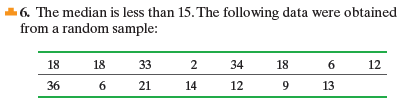×
Get Full Access to Statistics: Informed Decisions Using Data - 5 Edition - Chapter 15.3 - Problem 6
Get Full Access to Statistics: Informed Decisions Using Data - 5 Edition - Chapter 15.3 - Problem 6

×

# ?In Problems 1–6, use the sign test to test the given alternative hypothesis at the = 0.05 level of significance. 6. The median is less thanISBN: 9780134133539 240

## Solution for problem 6 Chapter 15.3

Statistics: Informed Decisions Using Data | 5th Edition

• Textbook Solutions
• 2901 Step-by-step solutions solved by professors and subject experts
• Get 24/7 help from StudySoup virtual teaching assistantsStatistics: Informed Decisions Using Data | 5th Edition

4 5 1 268 Reviews
24
5
Problem 6

In Problems 1–6, use the sign test to test the given alternative hypothesis at the= 0.05 level of significance.

6. The median is less than 15. The following data were obtained from a random sample:Step-by-Step Solution:

Step 1 of 5) In Problems 1–6, use the sign test to test the given alternative hypothesis at the = 0.05 level of significance. Figure 13(a) shows the histogram of the 2000 sample proportions from column p-hat. Notice that the shape of the distribution is skewed left. The mean of the 2000 sample proportions is 0.76 and the standard deviation is 0.136. Notice that the mean of the sample proportions equals the population proportion. Figure 13(b) shows the histogram for 2000 sample proportions from samples of size n = 25. Notice that the histogram is slightly skewed left (although not as skewed as the histogram with n = 10). The mean of the 2000 sample proportions for a sample of size n = 25 is 0.76 and the standard deviation is 0.086. Figure 13(c) shows the histogram for 2000 sample proportions from samples of size n = 60. Notice that the histogram is approximately normal. The mean of the 2000 sample proportions is 0.76 and the standard deviation is 0.054. Notice the following points regarding the sampling distribution of the sample proportion: Shape:

Step 2 of 2

## Discover and learn what students are asking

Statistics: Informed Decisions Using Data : Inference about Two Population Standard Deviations
?In Problems 9–14, assume that the populations are normally distributed. Test the given hypothesis. 11. s1 7 s2 at the a = 0.01 level of signi

Unlock Textbook Solution